Linear Ordinary Differential Equations of First and Second Order - 4

# Linear Ordinary Differential Equations of First and Second Order - 4 - Notes | Study Physics for IIT JAM, UGC - NET, CSIR NET - Physics

 1 Crore+ students have signed up on EduRev. Have you?

Second Order Linear Equations

The general form of these equations is

a2(t)y′′ + a1 (t)y′ + a0(t)y = b(t),

where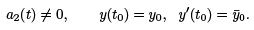If b(t) ≡ 0, we call it homogeneous. Otherwise, it is called non-homogeneous.

Homogeneous equations with constant coeﬃcients

This is the simplest case: a2, a1 , a0 are all constants, and g = 0. Let’s write: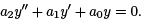Example 1. Solve y′′ − y = 0, (we have here a2 = 1, a1 = 0, a0 = 1).

Answer. Let’s guess an answer of the form y1(t) = et .

Check to see if it satisﬁes the equation: y′′ = e, so y′′ − y = et − e= 0. So it is a solution.

Guess another function: y2(t) = e−t .

Check:  y′ = −e−t , so y′′ = e−t, so y′′ − y = et − et = 0.

So it is also a solution.

Claim: Another function y = c1 y1 + c2 y2 for any arbitrary constants c1 , c2 (this is called “a linear combination of y1, y2 ”) is also a solution.

Check if this claim is true:

y(t) = c1e+ c2 e−t ,

then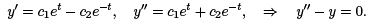Actually this claim is a general property. It is called the principle of superposition.

Theorem (The Principle of Superposition) Let y1(t) and y2(t) be solutions of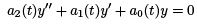Then, y = c1 y1 + c2 y2 for any constants c1 , c2 is also a solution.

Proof: If y1 solves the equation, then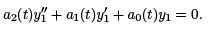(I)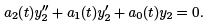(II)

Multiple (I) by c1 and (II) by c2, and add them up: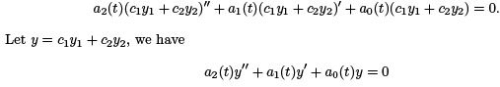therefore y is also a solution to the equation.

How to ﬁnd the solutions of

a2 y′′ + a1y′ + a0y = 0?

We seek solutions in the form y(t) = ert. Find r.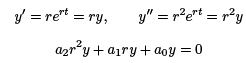Since y ≠ 0, we get

a2r2 + a1r + a0 = 0

This is called the characteristic equation.

Conclusion: If r is a root of the characteristic equation, then y = ert is a solution.
If there are two real and distinct roots r1 ≠ r2 , then the general solution is y(t) =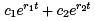where c1 , c2 are two arbitrary constants to be determined by initial conditions (ICs).

Example 2. Consider y′′ − 5y′ + 6y = 0.

(a). Find the general solution.

(b). If ICs are given as: y(0) = −1, y′ (0) = 5, ﬁnd the solution.

(c). What happens to y(t) when t → ∞?

Answer.(a). The characteristic equation is: r2 − 5r + 6 =, so (r − 2)(r − 3) = 0, two roots: r1 = 2, r2 = 3.

General solution is: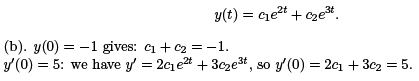Solve these two equations for c1, c2 : Plug in c2 = −1 − c1 into the second equation, we get 2c1 + 3(−1 − c1 ) = 5, so c1 = −8. Then c2 = 7. The solution is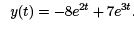(c). We see that y(t) = e2t · (−8 + 7e), and both terms in the product go to inﬁnity as t grows. So y → +∞ as t → +∞.

Example 3. Find the solution for 2y′′ + y′ − y = 0, with initial conditions y(1) = 0, y′(1) = 3.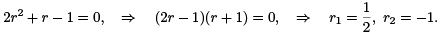.General solution is: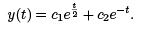The ICs give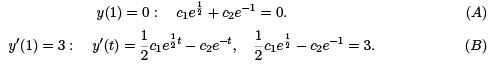(A)+(B) gives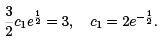Plug this in (A):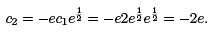The solution is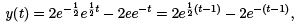and as t → ∞ we have y → ∞.

Example 4. Consider the equation y′′ − 5y = 0. (a).

Find the general solution.

(b). If the initial conditions are given as y(0) = 1 and y′(0) = a, then, for what values of a would y remain bounded as t → +∞?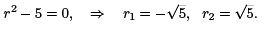General solution is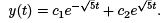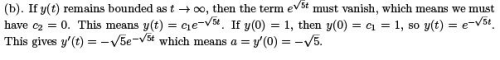Example 5. Consider the equation 2y′′ + 3y′ = 0. The characteristic equation is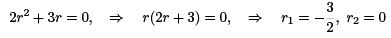The general solutions is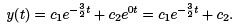As t → ∞, the ﬁrst term in y vanished, and we have y → c2 .

Example 6. Find a 2nd order equation such that c1 e3t + c2e−t is its general solution.

From the form of the general solution, we see the two roots are r1 = 3, r2 = −1.

The characteristic equation could be (r − 3)(r + 1) = 0, or this equation multiplied by any non-zero constant. So r2 − 2r − 3 = 0, which gives us the equation

y′′ − 2y′ − 3y = 0.

Solutions of Linear Homogeneous Equations; the Wronskian

We consider some theoretical aspects of the solutions to a general 2nd order linear equations.

Theorem .
(Existence and Uniqueness Theorem) Consider the initial value problem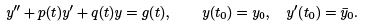If p(t), q(t) and g(t) are continuous and bounded on an open interval I containing t0, then there exists exactly one solution y(t) of this equation, valid on I .

Example 1. Given the equation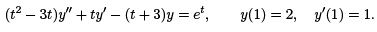Find the largest interval where solution is valid.

Answer. Rewrite the equation into the proper form: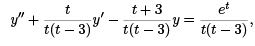so we have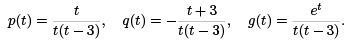We see that we must have t ≠ 0 and t ≠ 3. Since t0 = 1, then the largest interval is I = (0, 3), or 0 < t < 3. See the ﬁgure below.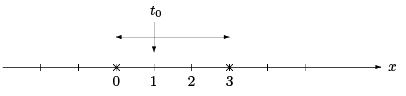Deﬁnition. Given two functions f (t), g(t), the Wronskian is deﬁned as

W (f, g)(t) =˙ f g′ − f ′g.

Remark: One way to remember this deﬁnition could be using the determinant of a 2 × 2 matrix,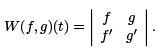Main property of the Wronskian:

• If W (f , g) ≡ 0, then f and g are linearly dependent.
• Otherwise, they are linearly independent.

Example 2. Check if the given pair of functions are linearly dependent or not.
(a). f = et , g = e−t .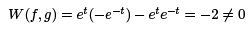so they are linearly independent. (b). f (t) = sin t, g(t) = cos t.

W (f , g) = sin t(sin t) − cos t cos t = −1 = 0

and they are linearly independent. (c). f (t) = t + 1, g(t) = 4t + 4.

Answer.We have W (f , g) = (t + 1)4 − (4t + 4) = 0

so they are linearly dependent. (In fact, we have g(t) = 4 · f (t).)

(d). f (t) = 2t, g(t) = |t|.

Answer. Note that g′ (t) = sign(t) where sign is the sign function. So

W (f , g) = 2t · sign(t) − 2|t| = 0

(we used t · sign(t) = |t|). So they are linearly dependent.

Theorem . Suppose y1(t), y2 (t) are two solutions of y′′ + p(t)y′ + q(t)y = 0.

Then

(I) We have either W (y1, y2 ) ≡ 0 or W (y1, y2) never zero;

II) If W (y1, y2) = 0, the y = c1 y1 + c2y2 is the general solution. They are also cal led to form a fundamental set of solutions. As a consequence, for any ICs y(t0) = y0,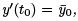there is a unique set of (c1 , c2 ) that gives a unique solution.

The next Theorem is probably the most important one.

Theorem (Abel’s Theorem) Let y1, y2 be two (linearly independent) solutions to
y′′ + p(t)y′ + q(t)y = 0
on an open interval I . Then, the Wronskian W (y1, y2) on I is given by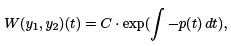for some constant C depending on y1, y2, but independent on t or on I .

Example 3. Given

t2 y′′ − t(t + 2)y′ + (t + 2)y = 0.

Find W (y1, y2) without solving the equation.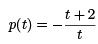which is valid for t ≠ 0. By Abel’s Theorem, we have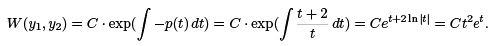NB! The solutions are deﬁned on either (0, ∞) or (−∞, 0), depending on t0.

From now on, when we say two solutions y1, yof the solution, we mean two linearly independent solutions that can form a fundamental set of solutions.

Example 4. If y1, y2 are two solutions of

ty′′ + 2y′ + tet y = 0,

and W (y1, y2)(1) = 2, ﬁnd W (y1, y2 )(5).

Answer. First we ﬁnd that p(t) = 2/t. By Abel’s Theorem we have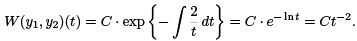If W (y1, y2)(1) = 2, then C = 2. So we have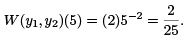Example 5. If W (f , g) = 3e4t , and f = e2t , ﬁnd g.

Answer.By deﬁnition of the Wronskian, we have

W (f , g) = f g′ − f ′g = e2t g′ − 2e2t g = 3e4t ,

which gives a 1st order equation for g:

g′ − 2g = 3e2t .

Solve it for g, by method of integrating factor :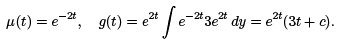We can choose c = 0, and get g(t) = 3te2t .

Next example shows how Abel’s Theorem can be used to solve 2nd order diﬀerential equations.

Example 6. Consider the equation y′′ + 2y′ + y = 0. Find the general solution.
The characteristic equation is r2 + 2r + 1 = 0, which given double roots r1 = r2 = −1. So we know that y1 = e−t is a solutions. How can we ﬁnd another solution y2 that’s linearly independent?

By Abel’s Theorem, we have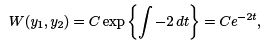and we can choose C = 1 and get W (y1, y2) = e−2t . By the deﬁnition of the Wronskian, we have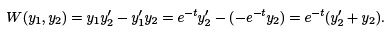These two computation must have the same answer, so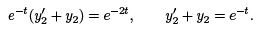This is a 1st order equation for y2. Solve it: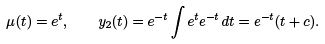Choosing c = 0, we get y2 = te. The general solution is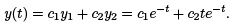This is called the method of reduction of order.

Complex Roots

Example : Consider the equation y′′ + y = 0, ﬁnd the general solution.

Answer. By inspection, we need to ﬁnd a function such that y′′ = −y. We see that y1 = cos t and y= sin t both work. By the Wronskian W (y1, y2 ) = −2 ≠ 0, we see that these two solutions are linearly independent.

Therefore, the general solution is
y(t) = c1 y1 + c2 y2 = c1 cos t + c2 sin t.

Let’ try to connect this with the characteristics equation:
r2 + 1 = 0, r2 = −1, r1 = i, r2 = −i.

The roots are complex. In fact, they are acomplex conjugate pair. We see that the imaginary part seems to give sin and cos functions.

In general, the roots of the characteristic equation can be complex numbers. Consider the equation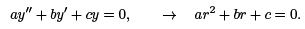The two roots are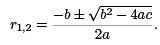If b2 − 4ac < 0, the root are complex, i.e., a pair of complex conjugate numbers. We will write r1,2 = λ ± iµ. There are two solutions: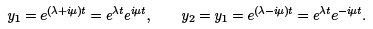To deal with exponential function with pure imaginary exponent, we need the Euler’s Formula: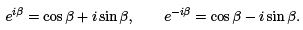Back to y1, y2 , we have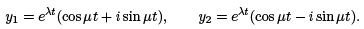But these solutions are complex-valued. We want real-valued solutions! To achieve this, we use the Principle of Superposition. If y1, y2 are two solutions, then cy1 + c2 y2 is also a solution for any constants c1 , c2 . In particular, the functions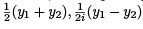are also solutions.
Write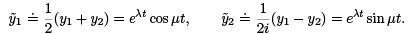We need to make sure that they are linearly independent. We can check the Wronskian,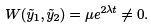(home work problem).

So y1, y2 are linearly independent, and we have the general solution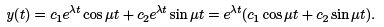Example 1. (Perfect Oscillation: Simple harmonic motion.) Solve the initial value problem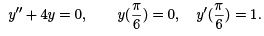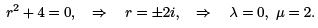The general solution is

y(t) = c1 cos 2t + c2 sin 2t.

Find c1 , c2 by initial conditions: since y′ = −2c1 sin 2t + 2c2 cos 2t, we have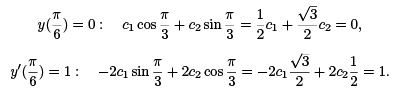Solve these two equations, we get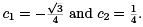So the solution is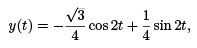which is a periodic oscillation. This is also called perfect oscillation or simple harmonic motion.

Example 2. (Decaying oscillation.) Find the solution to the IVP (Initial Value Problem)

y′′ + 2y′ + 101y = 0, y(0) = 1, y′(0) = 0.

r2 + 2r + 101 = 0, ⇒ r1,2 = −1 ± 10i, ⇒ λ = −1, µ = 10.

So the general solution is

y(t) = e−t(c1 cos 10t + c2 sin 10t),

so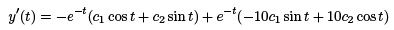Fit in the ICs: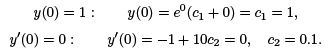Solution is

y(t) = e−t (cos t + 0.1 sin t).

The graph is given below: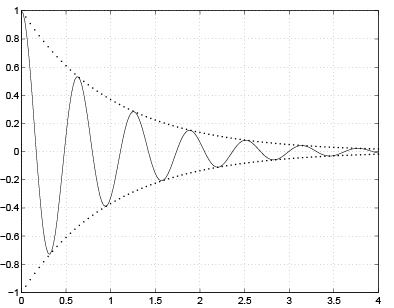We see it is a decaying oscillation. The sin and cos part gives the oscillation, and the e−t part gives the decaying amplitude. As t → ∞, we have y → 0.

Example 3. (Growing oscillation) Find the general solution of y′′ − y′ + 81.25y = 0.

Answer.  r2 − r + 81.25 = 0, ⇒ r = 0.5 ± 9i, ⇒ λ = 0.5, µ = 2.

The general solution is y(t) = e0.5t (ccos 9t + c2 sin 9t).

A typical graph of the solution looks like: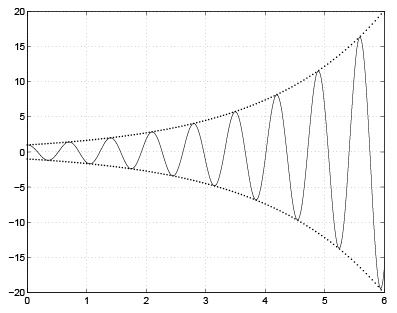We see that y oscillate with growing amplitude as t grows. In the limit when t → ∞, y oscillates between −∞ and +∞.

Conclusion: Sign of λ, the real part of the complex roots, decides the type of oscillation:

•  λ = 0: perfect oscillation;
• λ < 0: decaying oscillation;
• λ > 0: growing oscillation.

We note that since λ = $$-b \over 2a$$ , so the sign of λ follows the sign of −b/a.

The document Linear Ordinary Differential Equations of First and Second Order - 4 - Notes | Study Physics for IIT JAM, UGC - NET, CSIR NET - Physics is a part of the Physics Course Physics for IIT JAM, UGC - NET, CSIR NET.
All you need of Physics at this link: Physics

## Physics for IIT JAM, UGC - NET, CSIR NET

159 docs
 Use Code STAYHOME200 and get INR 200 additional OFF

## Physics for IIT JAM, UGC - NET, CSIR NET

159 docs

Track your progress, build streaks, highlight & save important lessons and more!

,

,

,

,

,

,

,

,

,

,

,

,

,

,

,

,

,

,

,

,

,

,

,

,

,

,

,

;